### Home > CALC > Chapter 6 > Lesson 6.1.4 > Problem6-53

6-53.
1. Evaluate. Homework Help ✎

1.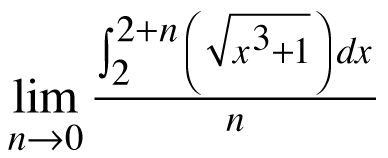2.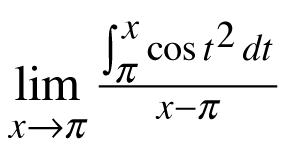3.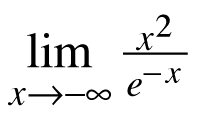4.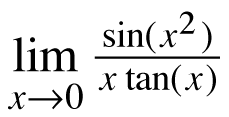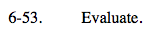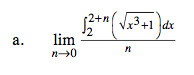Be careful! Evaluating this limit as n → 0 leads us to trouble: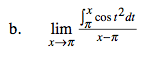Refer to part (a).

$\lim_{n\rightarrow 0}\frac{\int_{2}^{2+0} \left(\sqrt{x^{3}+1}\right)dx}{n}=\frac{0}{0}\text{ Recall that }\frac{0}{0}\text{ is an indeterminate form.}$

That means we do not know if the limit is finite (because the 0's 'cancel out') or infinite (because there is a 0 in the denominator).

We can evaluate an indeterminate limit if that limit happens to be a definition of the derivative.
Sure enough, part (a) is Ana's Definition of the Derivative:

Since n → 0, notice that a = 0. So we can rewrite the limit as:

So, we just need to find f '(x) at x = 0 and we have evaluated the limit.

Now evaluate that derivative at x = 0: